# Faculty and Researchers

## Mathematics Course, Department of Science

An introduction to faculty staff members and laboratories in the Mathematics Course, Department of Science.
Note: Information on the laboratories is current as of the 2023 academic year. There may be changes to this information in the 2024 academic year.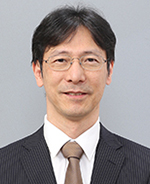#### IKEDA Toru

Position
Professor
Laboratory
Manifold Laboratory
##### Topology, 3D Manifolds, Knot Theory

A 3-manifold is a space in which a 3D coordinate system can be drawn around arbitrary points. Although it’s difficult to visualize an entire 3-manifold, work here involves cutting and pasting spaces and curves in order to study geometric properties such as symmetry.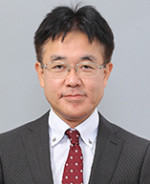#### ODA Fumihito

Position
Professor
Laboratory
Group Theory Laboratory
##### Representation Theory of Finite Groups

Examination is carried out into finite groups and their representation using a category theory approach. Research is conducted into group actions on simplicial complexes, semi-ordered sets, and modules.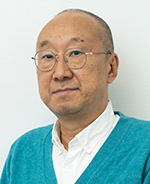#### SAKUMA Kazuhiro

Position
Professor
Laboratory
Topology Laboratory
##### Topology

I am studying 4D manifolds and the higher dimensional manifolds by using singularity theory of differentiable maps. There appears singular points in general which degenerate for the differential of smooth maps, so we try to observe the method of eliminating singularities with higer coranks.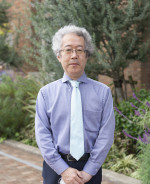#### CHINEN Koji

Position
Professor
Laboratory
Applicable Algebra Laboratory
##### Analytic Number Theory, Coding Theory

Coding theory is about ways to properly relay information. It is a comprehensive theory that is the result of numerous mathematical developments. Research is conducted with a focus on algebra, including coding theory as a mathematical theory and related group theory, and ring theory. Research is also conducted into analytic number theory as it relates to ciphers.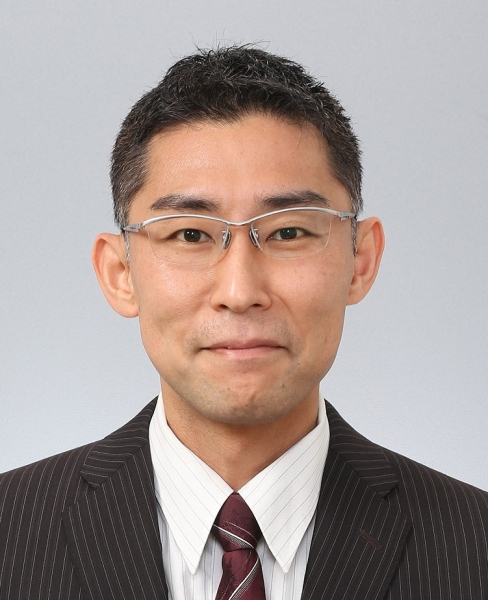#### MATSUI Yutaka

Position
Professor
Laboratory
Microlocal Analysis Laboratory
##### Mathematics, Algebraic Analysis, Microlocal Analysis

The field of specialty here is algebraic analysis, which grew out of research into differential equations. Using combinatorics and microlocal analysis, research is conducted into reversion formulas of radon transforms of constructible functions, and the behavior of images.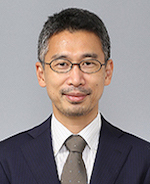#### YAMASHITA Tomoki

Position
Professor
Laboratory
Discrete Mathematics Laboratory
##### Graph Theory

Most discrete structures can be expressed in graph form. Graph theory represents a major research field of discrete mathematics. Here, research is conducted into extremal graph theory, which is the relationship between invariants and local substructures of the graph.#### ASAI Tsunenobu

Position
Associate Professor
Laboratory
Combinatorics Laboratory
##### Finite Group Theory

Research is conducted into finite groups and combinatorial structures constructed from groups.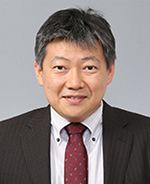#### IHARA Kentarou

Position
Associate Professor
##### Number Theory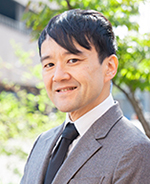#### SUZUKI Takao

Position
Associate Professor
Laboratory
Special Functions Laboratory
##### Special functions and Integrable systems

My research field is the theory of special functions which are defined by differential or difference equations in complex domains. These special functions are related to various fields of pure mathematics and applied mathematics. Therefore they are very interesting research object.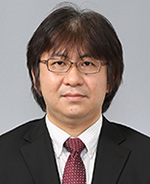#### In Dae JONG

Position
Associate Professor
Laboratory
Knot Theory Laboratory
##### Knot Theory

Research is conducted into knot theory and low-dimension topology (3D and 4D). To investigate knots mathematically, often the invariants of knots are used. Research takes an interest in the relation between the algebraic properties of invariants and the geometrical properties of knots.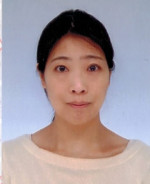#### NAKAMURA Yayoi

Position
Associate Professor
Laboratory
Computational Algebraic Analysis Laboratory
##### Computational Algebraic Analysis

By the usage of residues, multiple polylogarithms and multiple zeta values are studied.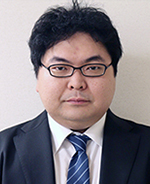#### ENDO Kazushige

Position
Assistant Professor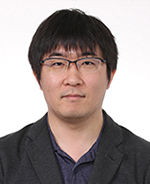#### KOMORI Daichi

Position
Assistant Professor